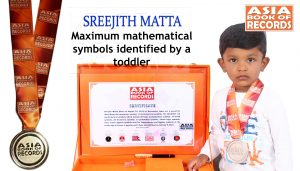# MAXIMUM MATHEMATICAL SYMBOLS IDENTIFIED BY A TODDLERSreejith Matta (born on August 23, 2018) of Karnataka, India, set a record for identifying the maximum number of mathematical symbols. He identified 112 mathematical symbols which include 28 basic mathematical symbols, 17 Greek symbols, 25 Geometry symbols, 23 probability symbols, eleven logic symbols, three Linear Algebra symbols and five Trigonometry and Algebra symbols, at the tender age of 2 years, 4 months and 7 days, as confirmed on January 8, 2021.